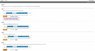# Find the final Celsius temperature of the aluminum and water

gamobigz

## Homework Statement

A 2.3kg aluminum pan at 135° is plunged into 3.0kg of water at 15°. The specific heat of aluminum is 900 J/(kg K).

Part A
Find the final Celsius temperature of the aluminum and water.
T_f = °Celsius

Part B
Find the entropy change of the aluminum pan.
ΔS_Al = J/K

Part C
Find the entropy change of the water.
ΔS_H20 = J/K

Part D
Find the entropy change in the system of particles consisting of all the particles in the aluminum plus all the particles in the water.
ΔS = J/K

Q=mCΔT
ΔS=Q/T

## The Attempt at a Solution

So my first couple attempts was that we use the equation Q=mC(T_f-T_i)

other related equations i know is DeltaS=Q/T but, how can i find the temperature final of both Water and aluminum.

I tried setting it up like this

m_water*C_water(T_f-T-i) + m_aluminum*C_aluminum(T_f-T_i) = Q_total

but i don't know how to get anything done from there.

Please help me with the first part and i should be able to most of it done on my own.

#### Attachments

•Entropy question.jpg
17.7 KB · Views: 436

gamobigz
I found the answer to the first two. And the first two will eventually help you with the rest.

attached in this form is a file showing the integration for part b.

Part A
Q_water+Qaluminum=0
m_waterC_water(T_f-T_water)+m_aluminumC_aluminum(T_f-T_aluminum)=0
using distribution from algebra and algebraically solving for T_F,
T_f=(m_waterC_waterT_water+m_aluminumCaluminumT_aluminum)/(m_waterC_water+m_aluminumC_aluminum)
you get Tf = 31.96 degrees Celsius.

m_aluminum=2.3kg
T_aluminum=135 degrees Celsius
C_aluminum=900J/kg*k
m_water=3kg
T_water=15 degrees celsius
C_water=4190J/kg*k

Part B

=(2.3kg)(900J/kg*k)ln((31.96+273)/(135+273))=-602.549J/K

Part C
use the same format, as b, but with different mass and specific heat, and T_i values, T_f is the same.

Part D is the sum of part B and C.

#### Attachments

Last edited:
Homework Helper
Gold Member
This all looks good.